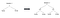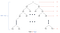# [Algorithm] 1. Growth of functions and Solving recurrences

The order of growth of running time of an algorithm is a convenient indicator that allows us to compare its performance with alternative algorithms and gives simplified information regarding how efficient such an algorithm is.

# 1. Definitions for the growth of functions with asymptotic notations

• what are asymptotic notations?

Asymptotic notations are languages that allow us to analyze an algorithm’s running time, which simply means how fast the algorithm is for given size of input, by identifying its behavior as the size of input grows. In this article, we are going to address 5 asymptotic notations which are 𝚶, 𝛀, 𝚯, 𝜊, 𝜔.

The notation 𝒏 often denotes the size of input, c implies some real constant, and 𝑓, 𝒈 are functions such that 𝑓, 𝒈: ℕ -> ℝ\{0}. In the below cases, for simplicity, we assume that both of 𝑓, 𝒈 are not zero.

• 1.1 Big-O 𝑓 ∈ 𝚶(𝒈):
• 1.2 Big-Omega 𝑓 ∈ 𝛀(𝒈):
• 1.3 Theta 𝑓 ∈ 𝚯(𝒈):
• 1.4 Small-o 𝑓 ∈ 𝜊(𝒈):
• 1.5 Small-Omega 𝑓 ∈ 𝜔(𝒈):

# 2. Solving recurrences

Recurrence relations, such as T(n) = 2T(n/2) + n, reflect the running time of such a recursive algorithm. Typically recurrence relations like the above example appear in divide-and-conquer algorithms since 2T(n/2) implies two subproblems of the size n/2 made by recursive call and n denotes the cost of combining sublists.

The following paragraphs will be dealing with the methods of how to solve these recurrence relations in order to get bounds on the runtime.

• 2.1 Substitution method

This is a way to solve recurrences with an appropriate guess. In other words, this method involves guessing the form of a solution for the given recurrence relation. This is used to find an upper or a lower bound. In this method for resolving recurrence relations, we take the following steps.

(1) Guess the form of the solution

(2) Use mathematical induction to find the constants and verify that the solution works

Various example for other recurrence relation can be found in the following link: http://itfs.egloos.com/m/9663923

• 2.2 Recursion tree

A recursion tree is a tree whose each node represents the cost of a certain recursive sub-problem. In order to get the cost of the entire algorithm, we need to sum up the costs in each node.

One of the strengths of the recursion tree is that it is useful to visualize what happens when a recurrence is iterated. Its diagram shows the structure of recursive calls, which means that how the main-problem divided into sub-problems, and the costs for combining those sub-tasks.

Figure 1 and 2 illustrate the recursion tree of the merge sort with its recurrence relation T(n) =2T(n/2)+nFigure 1. Recursion tree of the merge sort in recursive iteration step 1 and 2Figure 2. Complete recursion tree of the merge sort: T(n) = 2T(n/2) + n

Figure 3 shows the proof that T(n) ∈ 𝚶(𝒏log𝒏)

• note

(1) The depth of the recursion tree is log base b of n when the recurrence is in the form of T(n) = aT(n/b)+f(n)

(2) The depth of the recursion tree means the length of the longest branch among its branches.

For example) When recurrence is T(n) = T(n/3) + T(2n/3) + O(n), What is the depth of this recursion tree?

Refer 3.2 paragraph of the following link:https://web.stanford.edu/class/archive/cs/cs161/cs161.1168/lecture3.pdf

• 2.3 Master theorem

The explanation in the following link substitutes this part since I haven’t fully understood the master theorem.

https://en.wikipedia.org/wiki/Master_theorem_(analysis_of_algorithms)

# 3. Reference

Any corrections, suggestions, and comments are welcome

## jun-devpBlog

This publication is for organizing and posting what I’ve…

## jun-devpBlog

This publication is for organizing and posting what I’ve studied in scope of CS and Mathematics

Written by

## Jun

Novice Developer, Research Assistant in Fraunhofer IAIS, Master student of RWTH## jun-devpBlog

This publication is for organizing and posting what I’ve studied in scope of CS and Mathematics